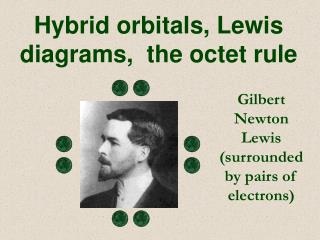DownloadDownload PresentationGilbert Newton Lewis (surrounded by pairs of electrons)

# Gilbert Newton Lewis (surrounded by pairs of electrons)

Télécharger la présentation## Gilbert Newton Lewis (surrounded by pairs of electrons)

- - - - - - - - - - - - - - - - - - - - - - - - - - - E N D - - - - - - - - - - - - - - - - - - - - - - - - - - -
##### Presentation Transcript

1. Hybrid orbitals, Lewis diagrams, the octet rule Gilbert Newton Lewis (surrounded by pairs of electrons)

2. Review • We can visualize a covalent bond as a balance between attraction and repulsion • A second way to view the formation of a bond is to consider orbital diagrams • Video (15 min - stop at HCl) • Now we can visualize atoms bonding because of the lower energy achieved via the stability of filled valence shells • The bottom line: atoms gain, lose, or share electrons to obtain noble gas electron configurations

3. Video: filling subshells

4. Video: filling subshells • Draw orbital diagrams for F+F, H + O, Li+F

5. 1s 1s 2s 2s 2p 2p 1s 1s 2p 2p 2s 2s 1s 1s 1s 2s Overlapping orbitals • Draw orbital diagrams for F+F, H + O, Li+F F2 H2O LiF is ionic (metal + non-metal)

6. Hybrid orbitals • Two overlapping orbitals form what is known as a hybrid or molecular orbital • Just as in a s,p,d, or f orbital the electrons can be anywhere in the orbital (even though the electron has started out in one atom, at times, it may be closer to the other nucleus) • Each hybrid orbital has a specific shape (described in chapter 8) • You do not need to know shapes • You need to know that hybrid orbitals exist and that they are formed from overlapping orbitals

7. Lewis diagrams • Read Pg. 229. Draw Lewis dot diagrams for Ne, Sb, Rb, F. How many variations of the Lewis diagram for P can be drawn? Ne Sb Rb F P P P P • Lewis diagrams follow the octet rule: atoms when forming ions, or bonding to other atoms in compounds have 8 outer electrons • Q - How can the octet rule be explained? • A - s (2 e–) and p (6 e–) orbitals are filled

8. e– 1) 2) Na Na+ Cl Cl– Cl– Na+ Ionic bonding • Recall: Ionic bonding involves 3 steps: 1) loss of e-, 2) gain of e-, 3) +ve, -ve attract 3) This can be represented via Lewis diagrams… • Read remainder of 7.2 (pg. 230) • Diagram the reaction between Li + Cl and Mg + O (PE 3)

9. O [Mg]2+ [ O ]2– Mg Cl [Li]+ [ Cl ]– Li The octet rule (ionic compounds) • Draw Li + Cl and Mg + O (PE 3, pg. 230) • Note also that the charge on an ion can be determined by the number of places removed from a noble gas (Ca, N, Al?) • Ca2+, N3-, Al3+ • See pg. 230 for example of Ca + Cl

10. Cl Cl C Cl Cl H H H H H Cl N H O [Mg]2+ [ F ]2– Covalent bonding • Covalent bonds can also be shown via Lewis diagrams - E.g draw Lewis diagrams showing the combination of 1) H+Cl, 2) C+Cl, 3) H+O, 4) Mg+F, 5) N+H, 6) Do PE 4 (pg. 234) H2O NH3 HCl MgF2 - Ionic CCl4 • Note bonds can also be drawn with a dash to represent two electrons (read 234-5 upto 7.5)

11. The octet rule (covalent compounds) • (Read 7.4 up to PE4 (pg. 232 - 4). Do PE4.) • Read remainder of 7.4 • Although the octet rule works for most compounds, PCl5 and SF6 are examples of exceptions (see pg. 235 for structures) • Also, it can be difficult to draw the correct bonds for atoms with multiple bonds • Some clear rules have been established for drawing Lewis structures • We will see that the rules dictate molecular structure, which dictates shape, which can influence chemical properties of a compound For more lessons, visit www.chalkbored.com